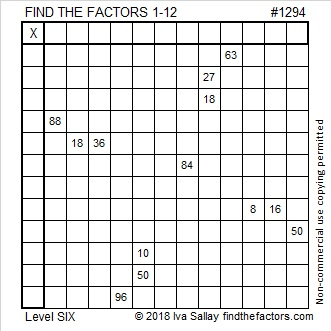# 1294 and Level 6

You will need to use multiplication facts from 1 to 12, logic, and perseverance to solve this tricky level 6 puzzle. Good luck!Print the puzzles or type the solution in this excel file: 12 factors 1289-1299

Here are some facts about the number 1294:

• 1294 is a composite number.
• Prime factorization: 1294 = 2 × 647
• The exponents in the prime factorization are 1 and 1. Adding one to each and multiplying we get (1 + 1)(1 + 1) = 2 × 2 = 4. Therefore 1294 has exactly 4 factors.
• Factors of 1294: 1, 2, 647, 1294
• Factor pairs: 1294 = 1 × 1294 or 2 × 647
• 1294 has no square factors that allow its square root to be simplified. √1294 ≈ 35.972211294 is also the sum of the twenty prime numbers from 23 to 107.